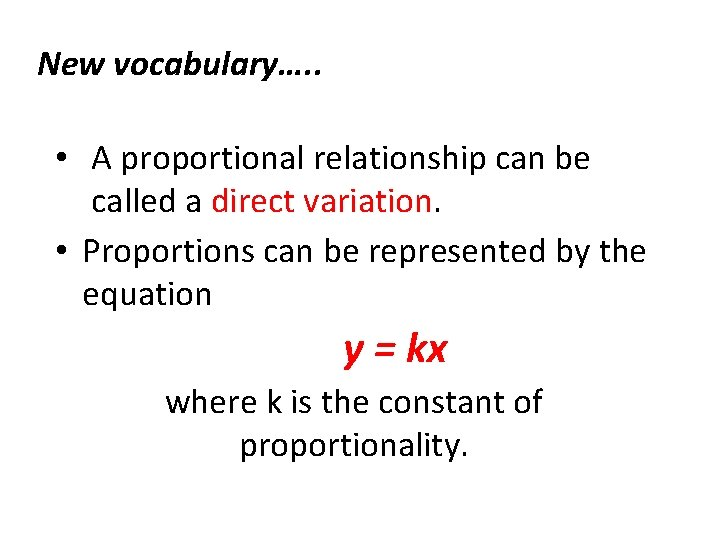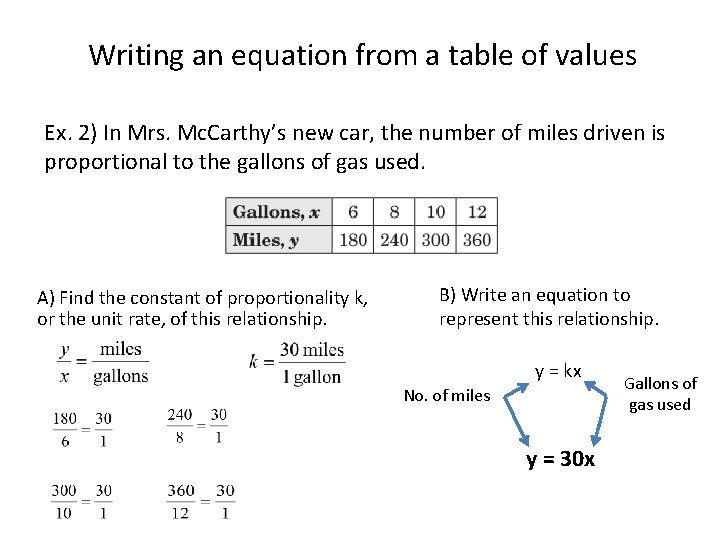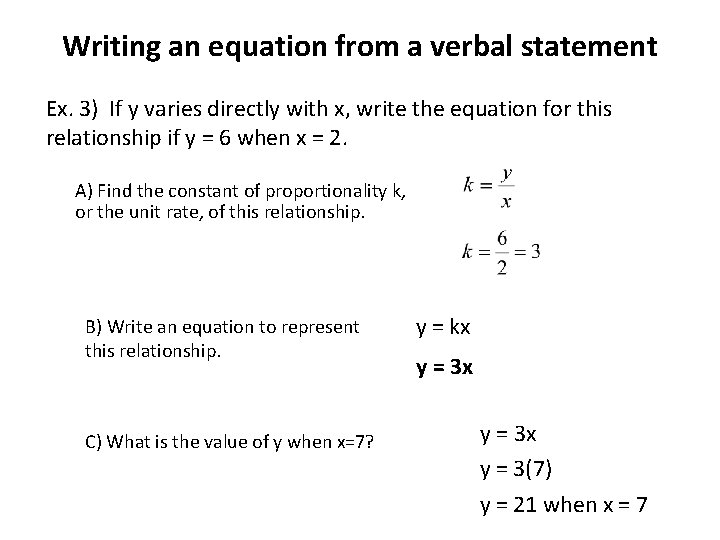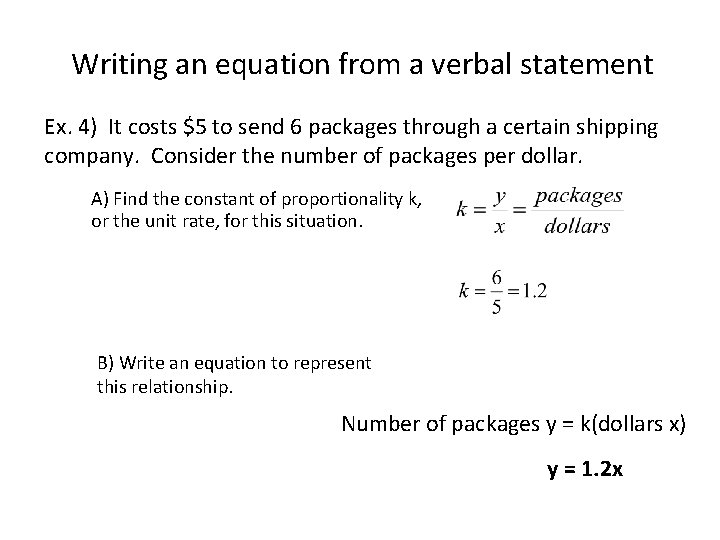# Equations of proportional relationships What weve learned Proportional

• Slides: 7Equations of proportional relationshipsWhat we’ve learned…. . • Proportional relationships have a constant ratio, or unit rate. • The constant ratio, or unit rate, can also be called the constant of proportionality. • The graph of a proportional relationship is a straight line that passes through the origin.New vocabulary…. . • A proportional relationship can be called a direct variation. • Proportions can be represented by the equation y = kx where k is the constant of proportionality.Writing an equation from the graph of a proportional relationship Ex. 1) The number of students that can go on a trip is proportional to the number of chaperones that are available. (4, 24) A) Find the constant of proportionality k, or the unit rate, of this relationship. (3, 18) (2, 12) (1, 6) B) Write an equation to represent this relationship. y = kx No. of students No. of chaperones y = 6 xWriting an equation from a table of values Ex. 2) In Mrs. Mc. Carthy’s new car, the number of miles driven is proportional to the gallons of gas used. A) Find the constant of proportionality k, or the unit rate, of this relationship. B) Write an equation to represent this relationship. y = kx No. of miles y = 30 x Gallons of gas usedWriting an equation from a verbal statement Ex. 3) If y varies directly with x, write the equation for this relationship if y = 6 when x = 2. A) Find the constant of proportionality k, or the unit rate, of this relationship. B) Write an equation to represent this relationship. C) What is the value of y when x=7? y = kx y = 3 x y = 3(7) y = 21 when x = 7Writing an equation from a verbal statement Ex. 4) It costs \$5 to send 6 packages through a certain shipping company. Consider the number of packages per dollar. A) Find the constant of proportionality k, or the unit rate, for this situation. B) Write an equation to represent this relationship. Number of packages y = k(dollars x) y = 1. 2 x